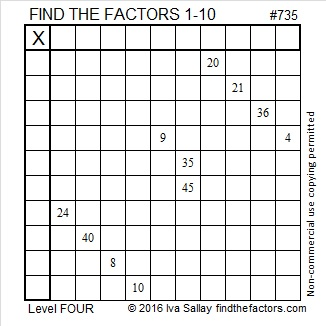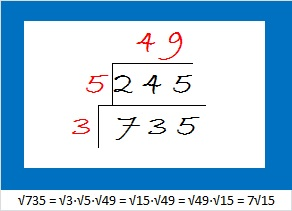# 735 and Level 4

• 735 is a composite number.
• Prime factorization: 735 = 3 x 5 x 7 x 7, which can be written 735 = 3 x 5 x (7^2)
• The exponents in the prime factorization are 1, 1, and 2. Adding one to each and multiplying we get (1 + 1)(1 + 1)(2 + 1) = 2 x 2 x 3 = 12. Therefore 735 has exactly 12 factors.
• Factors of 735: 1, 3, 5, 7, 15, 21, 35, 49, 105, 147, 245, 735
• Factor pairs: 735 = 1 x 735, 3 x 245, 5 x 147, 7 x 105, 15 x 49, or 21 x 35
• Taking the factor pair with the largest square number factor, we get √735 = (√49)(√15) = 7√15 ≈ 27.11088.Here’s a medium level factoring puzzle for you to try:Print the puzzles or type the solution on this excel file: 10 Factors 2016-01-04

———————————————————

735 = 7²·3·5Stetson.edu informs us that 735 is the smallest number whose digits and distinct prime factors are the same.

735 is the sum of consecutive numbers many different ways:

• 367 + 368 = 735; that’s 2 consecutive numbers.
• 244 + 245 + 246 = 735; that’s 3 consecutive numbers.
• 145 + 146 + 147 + 148 + 149 = 735; that’s 5 consecutive numbers.
• 120 + 121 + 122 + 123 + 124 + 125 = 735; that’s 6 consecutive numbers.
• 102 + 103 + 104 + 105 + 106 + 107 + 108 = 735; that’s 7 consecutive numbers.
• 69 + 70 + 71 + 72 + 73 + 74 + 75 + 76 + 77 + 78 = 735; that’s 10 consecutive numbers.
• 46 + 47 + 48 + 49 + 50 + 51 + 52 + 53 + 54 + 55 + 56 + 57 + 58 + 59 = 735; that’s 14 consecutive numbers.
• 42 + 43 + 44 + 45 + 46 + 47 + 48 + 49 + 50 + 51 + 52 + 53 + 54 + 55 + 56 = 735; that’s 15 consecutive numbers.
• 25 + 26 + 27 + 28 + 29 + 30 + 31 + 32 + 33 + 34 + 35 + 36 + 37 + 38 + 39 + 40 + 41 + 42 + 43 + 44 + 45 = 735; that’s 21 consecutive numbers.
• 10 + 11 + 12 + 13 + 14 + 15 + 16 + 17 + 18 + 19 + 20 + 21 + 22 + 23 + 24 + 25 + 26 + 27 + 28 + 29 + 30 + 31 + 32 + 33 + 34 + 35 + 36 + 37 + 38 + 39 = 735; that’s 30 consecutive numbers.
• 4  + 5 + 6 + 7 + 8 + 9 + 10 + 11 + 12 + 13 + 14 + 15 + 16 + 17 + 18 + 19 + 20 + 21 + 22 + 23 + 24 + 25 + 26 + 27 + 28 + 29 + 30 + 31 + 32 + 33 + 34 + 35 + 36 + 37 + 38 = 735; that’s 35 consecutive numbers.

Because 5 is one of its factors, 735 is the hypotenuse of Pythagorean triple 441-588-735. What is the greatest common factor of those three numbers? 147, the other half of the factor pair with 5.

735 is a palindrome in two other bases:

• 3223 BASE 6; note that 3(216) + 2(36) + 2(6) + 3(1) = 735.
• LL BASE 34 (L = 21 base 10); note that 21(24) + 21(1) = 735.

If I were calculating the square root of 735, I would make a little factor cake using only prime numbers and prime numbers squared on the outside of the cake, and then I would take the square root of everything on the outside of the cake:———————————————————